# Mathematical Functions¶

This chapter describes basic mathematical functions. Some of these functions are present in system libraries, but the alternative versions given here can be used as a substitute when the system functions are not available.

The functions and macros described in this chapter are defined in the header file cml/math.h.

## Mathematical Constants¶

The library ensures that the standard BSD mathematical constants are defined. For reference, here is a list of the constants:

 M_E The base of exponentials,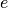M_LOG2E The base-2 logarithm of,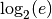M_LOG10E The base-10 logarithm of,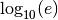M_SQRT2 The square root of two,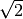M_SQRT1_2 The square root of one-half,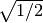M_SQRT3 The square root of three,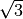M_PI The constant pi,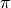M_PI_2 Pi divided by two,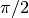M_PI_4 Pi divided by four,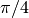M_SQRTPI The square root of pi,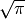M_2_SQRTPI Two divided by the square root of pi,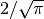M_1_PI The reciprocal of pi,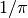M_2_PI Twice the reciprocal of pi,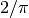M_LN10 The natural logarithm of ten,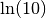M_LN2 The natural logarithm of two,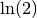M_LNPI The natural logarithm of pi,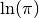M_EULER Euler’s constant,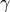## Infinities and Not-a-number¶

POSINF

This macro contains the IEEE representation of positive infinity,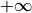. It is computed from the expression +1.0/0.0.

NEGINF

This macro contains the IEEE representation of negative infinity,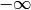. It is computed from the expression -1.0/0.0.

NAN

This macro contains the IEEE representation of the Not-a-Number symbol, NaN. It is computed from the ratio 0.0/0.0.

bool cml_isnan(double x)

This function returns 1 if x is not-a-number.

bool cml_isinf(double x)

This function returns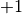if x is positive infinity,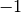if x is negative infinity and 0 otherwise. 

bool cml_isfinite(double x)

This function returns 1 if x is a real number, and 0 if it is infinite or not-a-number.

## Elementary Functions¶

The following routines provide portable implementations of functions found in the BSD math library. When native versions are not available the functions described here can be used instead. The substitution can be made automatically if you use autoconf to compile your application (see portability-functions).

double cml_log1p(double x)

This function computes the value of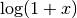in a way that is accurate for small x. It provides an alternative to the BSD math function log1p(x).

double cml_expm1(double x)

This function computes the value of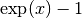in a way that is accurate for small x. It provides an alternative to the BSD math function expm1(x).

double cml_hypot(double x, double y)

This function computes the value of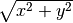in a way that avoids overflow. It provides an alternative to the BSD math function hypot(x,y).

double cml_hypot3(double x, double y, double cml_z)

This function computes the value of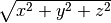in a way that avoids overflow.

double cml_acosh(double x)

This function computes the value of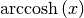. It provides an alternative to the standard math function acosh(x).

double cml_asinh(double x)

This function computes the value of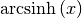. It provides an alternative to the standard math function asinh(x).

double cml_atanh(double x)

This function computes the value of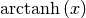. It provides an alternative to the standard math function atanh(x).

double cml_ldexp(double x, int e)

This function computes the value of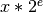. It provides an alternative to the standard math function ldexp(x,e).

double cml_frexp(double x, int *e)

This function splits the number x into its normalized fraction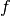and exponent, such that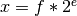and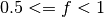. The function returnsand stores the exponent in. If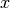is zero, bothandare set to zero. This function provides an alternative to the standard math function frexp(x, e).

## Small integer powers¶

A common complaint about the standard C library is its lack of a function for calculating (small) integer powers. CML provides some simple functions to fill this gap. For reasons of efficiency, these functions do not check for overflow or underflow conditions.

double cml_pow_int(double x, int n)
double cml_pow_uint(double x, unsigned int n)

These routines computes the power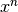for integer n. The power is computed efficiently—for example,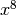is computed as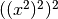, requiring only 3 multiplications.

double cml_pow_2(double x)
double cml_pow_3(double x)
double cml_pow_4(double x)
double cml_pow_5(double x)
double cml_pow_6(double x)
double cml_pow_7(double x)
double cml_pow_8(double x)
double cml_pow_9(double x)

These functions can be used to compute small integer powers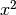,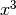, etc. efficiently. The functions will be inlined when HAVE_INLINE is defined, so that use of these functions should be as efficient as explicitly writing the corresponding product expression:

#include <cml.h>
[...]
double y = pow_4(3.141);  /* compute 3.141**4 */


## Testing the Sign of Numbers¶

double cml_sgn(double x)

This macro returns the sign of x. It is defined as ((x) >= 0 ? 1 : -1). Note that with this definition the sign of zero is positive (regardless of its IEEE sign bit).

## Maximum and Minimum functions¶

Note that the following macros perform multiple evaluations of their arguments, so they should not be used with arguments that have side effects (such as a call to a random number generator).

CML_MAX(a, b)

This macro returns the maximum of a and b. It is defined as ((a) > (b) ? (a):(b)).

CML_MIN(a, b)

This macro returns the minimum of a and b. It is defined as ((a) < (b) ? (a):(b)).

## Approximate Comparison of Floating Point Numbers¶

It is sometimes useful to be able to compare two floating point numbers approximately, to allow for rounding and truncation errors. The following function implements the approximate floating-point comparison algorithm proposed by D.E. Knuth in Section 4.2.2 of “Seminumerical Algorithms” (3rd edition).

bool cml_cmp(double x, double y, double epsilon)

This function determines whether x and y are approximately equal to a relative accuracy epsilon.

The relative accuracy is measured using an interval of size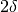, where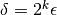and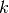is the maximum base-2 exponent ofand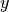as computed by the function frexp().

Ifandlie within this interval, they are considered approximately equal and the function returns 0. Otherwise if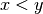, the function returns, or if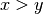, the function returns.

Note thatandare compared to relative accuracy, so this function is not suitable for testing whether a value is approximately zero.

The implementation is based on the package fcmp by T.C. Belding.

Footnotes

  Note that the C99 standard only requires the system isinf() function to return a non-zero value, without the sign of the infinity. The implementation in some earlier versions of CML used the system isinf() function and may have this behavior on some platforms. Therefore, it is advisable to test the sign of x separately, if needed, rather than relying the sign of the return value from isinf().